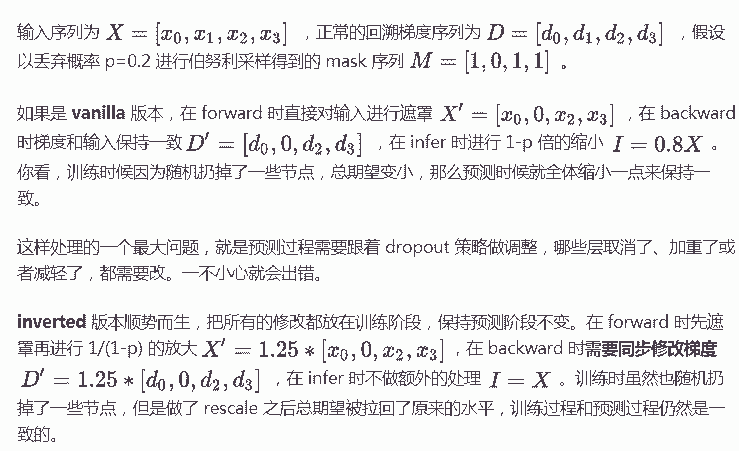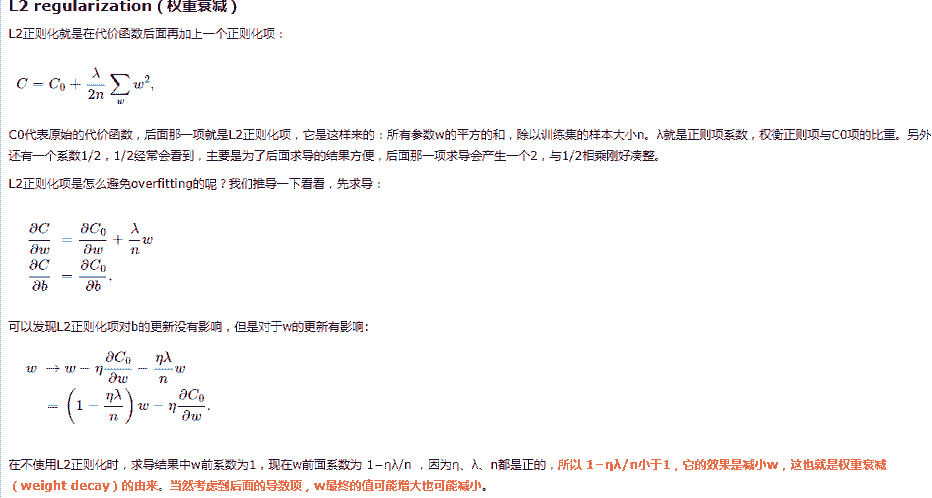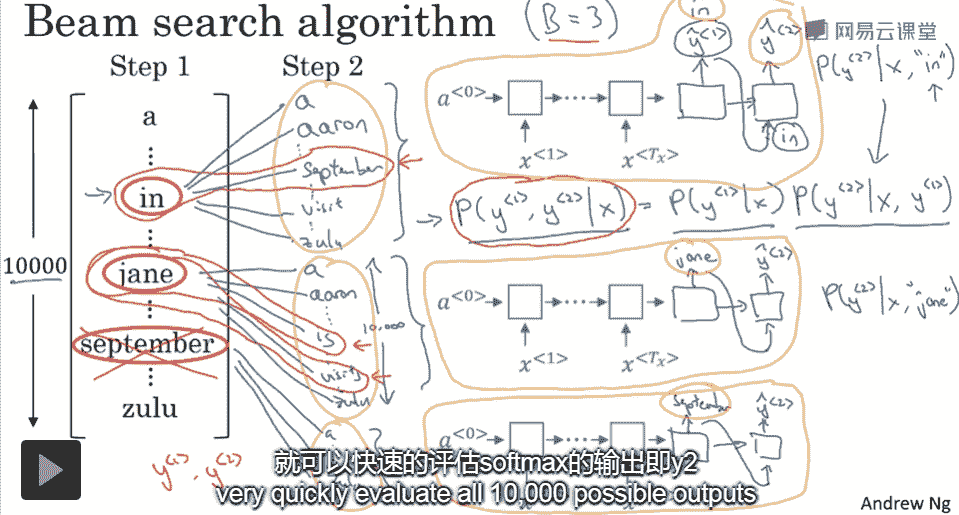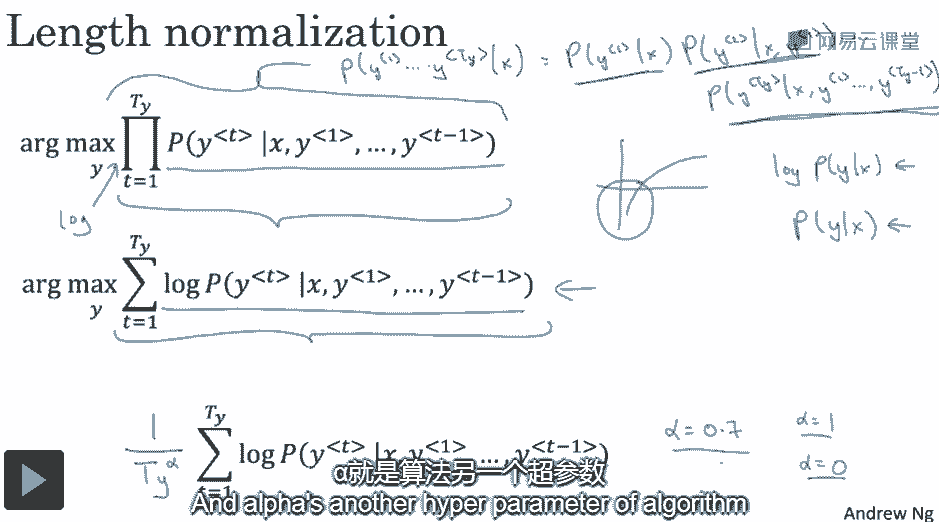# 塞进裤子ヾ(≧O≦)〃嗷~

0%

## 梯度消失与爆炸

Vanishing/Exploding Gardients

RNN梯度消失或爆炸原因。

https://zhuanlan.zhihu.com/p/28687529

LSTM如何解决(缓解)梯度消失的？

LSTM通过gate可以决定梯度消失的程度，比如让遗忘门接近于1，从而让这个时刻的信息一直传递下去而不丢失。

## Dropout

vanilla dropoutinverted dropout

inverted dropout指的是若以概率p丢弃神经元，前向传播的时候要先除以（1-p）以保持总期望不变。re：神经网络Dropout层中为什么dropout后还需要进行rescale？ - 小莲子的回答 - 知乎

## 过拟合overfitting

$\lambda$大的适合，w变得更小，$\lambda$小的适合，w变得更大。## Model

### logistic regression

$P(y=1|x) = sigmoid(W^Tx+b)=\frac{1}{1+e^{-W^Tx-b}}$

$$L(y,\hat y)=-[y^ilog \hat y^i+(1-y^i)log(1- \hat y^i)]$$

$$J(W,b) = \frac{1}{N}\sum_i^NL(yi, \hat yi)$$

### softmax regression

softmax regression将logistic regression(二分类)推广到多分类。

$P(y=1|x) = softmax(W^Tx+b)$

$$L(y,\hat y)=-y^ilog \hat y^i$$

$$Loss Function = \frac{1}{N}\sum_i^NL(yi, \hat yi)$$

### multi label learning

$y^i=\left[ \begin{matrix} 0 \ 1 \ 0 \ 0 \ 0 \end{matrix} \right]$

$y^i=\left[ \begin{matrix} 0 \ 1 \ 1 \ 1 \ 0 \end{matrix} \right]$

$$L(y^i_j,\hat y^i_j)=-[y^i_jlog \hat y^i_j+(1-y^i_j)log(1- \hat y^i_j)]$$

$$Loss Function = \frac{1}{N}\sum_i^N \sum_{j=1}^5 L(y^i_j, \hat y^i_j)$$beam width大，速度慢，效果好。beam width小，速度快，效果差。

### 改进beam search：length normalization

T是生成的句子长度，$\alpha$是超参数

# 问题

### softmax为什么采用指数

softmax目的是为了获得max的那个元素

if help:小手一抖点个广告 or 大手一挥资助一下